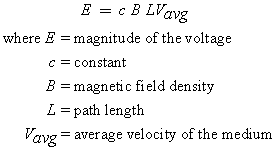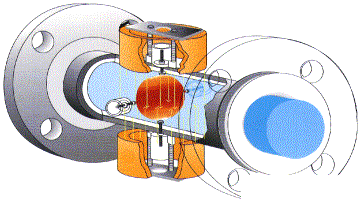# Magnetic Measuring Principle

## Magnetic Technology in Mass Flow Meters

Electromagnetic or Magnetic flowmeters are designed to measure the flow of electrically charged liquids in a closed pipe. They are volumetric flow measuring devices. With this technology, the fluid must be conductive. The principle of operation of the magnetic flowmeter is based on Michael Faraday’s Law of Electromagnetic Induction.

## Mass Flow Meter Governing  FormulaThe above equation indicates that a voltage is induced when a conductor (conductive fluid) is passed through a magnetic field. The voltage developed is proportional to the density of the magnetic field, the distance between electrodes, and the velocity of the conductive fluid moving through the field. The voltage is extracted through a pair of electrodes that are installed on opposite sides of the pipe. Special attention is required when the pipe is constructed of conductive material. A lining of nonconductive material is used to prevent the voltage from being dissipated into the pipe section.

The magnetic field density is fixed for each magnetic flowmeter size. Therefore, velocity is left as the only variable, which means that magnetic flowmeter is a velocity-measuring device.

### Should You Use a Magnetic Mass Flowmeter?

When choosing whether or not to use a magnetic mass flowmeter in a given application, the first parameter to consider is the electrical conductivity. Applications comprise conductive fluids (liquids and slurries). For a list of known fluids, SmartMeasurement™ has constructed a conductivity table.